﻿ 房价与GDP、货币供应周期波动及其关系研究 Cycle Fluctuation among Housing Price, GDP and Money Supply

Statistics and Application
Vol. 08  No. 02 ( 2019 ), Article ID: 29562 , 11 pages
10.12677/SA.2019.82029

Cycle Fluctuation among Housing Price, GDP and Money Supply

Tianbang Cao1, Ping Dong2,3, Yuqi Lu2,3*

1Jiangsu Jinningda Real Estate Planning Mapping Valuation Co., Ltd., Nanjing Jiangsu

2College of Geographic Science, Nanjing Normal University, Nanjing Jiangsu

3Jiangsu Center for Collaborative Innovation in Geographical Information Resource Development and Application, Nanjing Jiangsu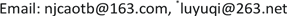Received: Mar. 11th, 2019; accepted: Mar. 26th, 2019; published: Apr. 2nd, 2019ABSTRACT

The study investigated the cyclical relationship among housing price, GDP and money supply, using single-spectral analysis and cross-spectral analysis. The results demonstrated that: 1) From the results of single-spectral analysis, during 1979-2016, there are some medium-term cycles of about 10 years and short cycles of about 5 years in the process of GDP growth. During 1991-2016, there are some short circles of about 3 years and medium-term cycles of about 9 years in the money supply growth. During 1993-2016, there are some short cycles of 2 - 3 years and medium-term cycles of about 12 years in the process of housing price growth. 2) During the period of 1993-2016, the growth of GDP, money supply M1 and house price growth all had a strong correlation in the two-year cycle, and the growth volatility of GDP and M1 was basically synchronized with the fluctuation of house price growth.

Keywords:Housing Price, GDP, Money Supply, Spectral Analysis

1江苏金宁达房地产评估规划测绘咨询有限公司，江苏 南京

2南京师范大学地理科学学院，江苏 南京

3江苏省地理信息资源开发与利用协同创新中心，江苏 南京Copyright © 2019 by authors and Hans Publishers Inc.

This work is licensed under the Creative Commons Attribution International License (CC BY).

http://creativecommons.org/licenses/by/4.0/1. 引言

2. 数据来源和研究方法

2.1. 数据来源

2.2. 研究方法

2.2.1. 单谱分析

${y}_{t}={A}_{0}+\underset{t=1}{\overset{n}{\sum }}\left(Am\mathrm{cos}2\text{π}mt/N+Bm\mathrm{sin}2\text{π}mt/N\right)+{\epsilon }_{t},\left(m=1,2,\cdots ,n\right)$ (1)Table 1. Gross domestic product, money supply and commercial housing sales price growth rate

${A}_{m}=\frac{1}{N}\underset{t=1}{\overset{N}{\sum }}{X}_{t}\mathrm{cos}2\text{π}mt/N,\left(m=1,2,\cdots ,n\right)$ (2)

${B}_{m}=\frac{1}{N}\underset{t=1}{\overset{N}{\sum }}{X}_{t}\mathrm{sin}2\text{π}mt/N,\left(m=1,2,\cdots ,n\right)$ (3)

$I\left(fm\right)=N\left({A}_{m}^{2}+{B}_{m}^{2}\right),\left(m=1,2,\cdots ,n\right)$ (4)

$\mathrm{max}\left\{N\left[{A}_{m}^{2}+{B}_{m}^{2}\right]\to N,N\left[{A}_{m}^{2}+{B}_{m}^{2}\right]\to \text{ }\text{ }\frac{N}{2}\text{ },\cdots ,\left[{A}_{m}^{2}+{B}_{m}^{2}\right]\to \text{ }\text{ }\frac{N}{n}\text{ }\text{ }\right\}$ (5)

2.2.2. 交叉谱分析

${C}_{xy}=\frac{1}{n-k}\underset{t=1}{\overset{n-k}{\sum }}\frac{{x}_{t}-\stackrel{¯}{x}}{{S}_{x}}×\frac{{y}_{t+k}-\stackrel{¯}{y}}{{S}_{y}}$ (6)

${C}_{yx}=\frac{1}{n-k}\underset{t=1}{\overset{n-k}{\sum }}\frac{{x}_{t+k}-\stackrel{¯}{x}}{{S}_{x}}×\frac{{y}_{t}-\stackrel{¯}{y}}{{S}_{y}}$ (7)

${P}_{j}=\left({B}_{j}/m\right){C}_{xy}\left(0\right)+\left(1/2\right)\underset{k=1}{\overset{m}{\sum }}{\lambda }_{k}\left[{C}_{xy}\left(k\right)+{C}_{yx}\left(k\right)\right]\mathrm{cos}\left(\text{π}jk/m\right)$ (9)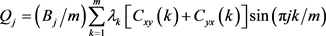(10)

${A}_{j}=\sqrt{{P}_{j}^{2}+{Q}_{j}^{2}}$ (11)

${H}_{j}=\mathrm{arctan}\left(-{Q}_{j}/{P}_{j}\right)$ (12)

${O}_{j}={A}_{j}^{2}/\left({S}_{xj}×{S}_{yj}\right)$ (13)

3. 研究结果分析

3.1. 数据预处理

3.1.1. 消除长期趋势

3.1.2. 平稳性检验

3.2. 单谱分析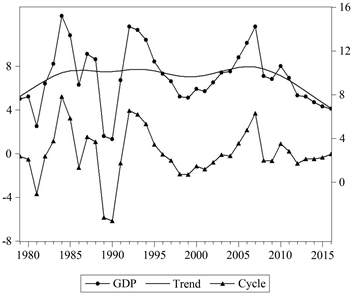Figure 1. National GDP growth rate and its periodic components after HP filtering decomposition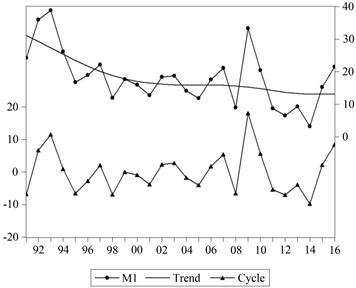Figure 2. National money supply M1 growth rate and its periodic components after HP filter decomposition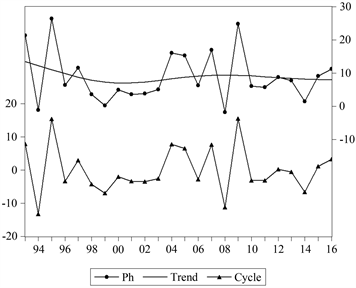Figure 3. The average sales price growth rate of commercial housing and the periodic components after HP filtering decompositionTable 2. Stationarity test of periodic component sequences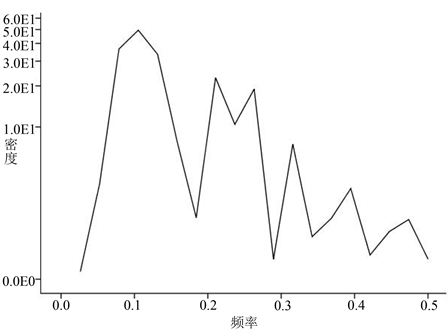Figure 4. National GDP growth sequence spectral density curve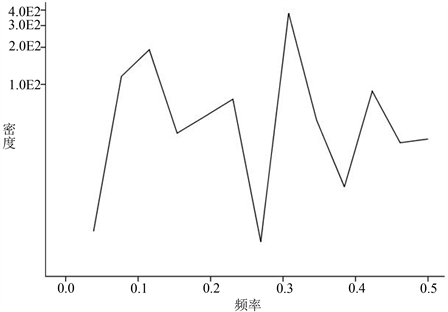Figure 5. National monetary supply M1 growth sequence spectral density curve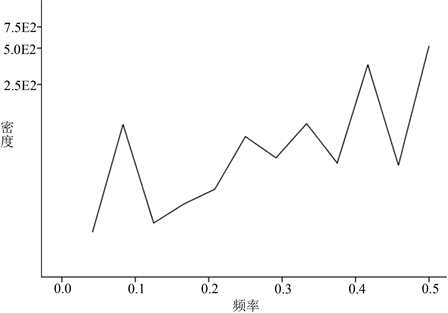Figure 6. National product housing average sales price growth sequence spectral density curve

3.3. 交叉谱分析

3.3.1. 结果分析Table 3. Cross-spectrum analysis of national GDP growth rate and Ph growth rate

ContinuedTable 4. Cross-spectrum analysis of national M1 growth rate and Ph growth rate

3.3.2. Granger因果关系检验Table 5. Test results of causality between GDP growth rate and Ph growth rateTable 6. Test results of causality between M1 growth rate and Ph growth rate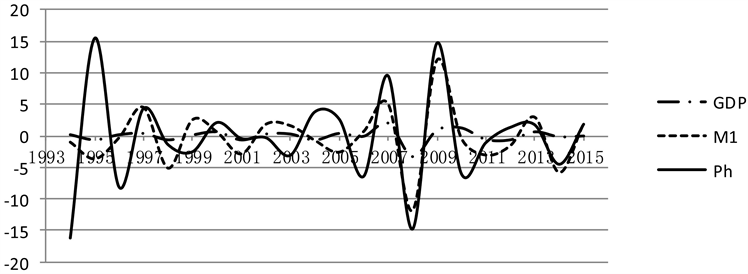注：对BP滤波带通宽度为PL = 2，PU = 8。

Figure 7. Cycle curve of GDP, M1 and commercial house sales price growth rate after BP filtering

3.3.3. 波动原因分析

1) 房价与GDP关系。经济发展意味着财政、金融景气，经济繁荣，社会总投资增加，生产活动活跃，对厂房、写字楼、商店、住宅和各种娱乐设施等的需求增加，由此会引起房地产价格上涨；相反经济停滞，需求减弱，房价下跌。

2) 房价与M1关系。① 信贷传导机制分析。房地产开发的资金需求量极大，银行贷款是其主要资金来源，银行贷款规模对房价影响至深。当中央银行实行宽松货币政策时，银行可贷资金增大，对房地产企业的资金供给增多，导致房地产供给增加。同时，居民个人住房贷款需求更易得到满足，自然刺激房价上涨。② 利率传导机制分析。从个人消费角度看，当货币供给增加时，利率水平下降，房屋需求上升，再加上按揭贷款的成本相对减少，居民还款能力提高，也进一步刺激其购房需求，推动房价上涨。从企业投资角度看，货币供给增加，利率下降，企业融资成本降低，投资的边际收益率提高，从而刺激投资增长。房地产企业将获得更大的资金回旋余地，利于其通过提价营销策略。相反收紧银根，房地产投资和消费需求将受到抑制，房价会下跌。

4. 结论与启示

4.1. 结论

1) 采用单谱分析，在1979~2016年期间，GDP增长有10年左右的中周期，还有5年左右的短周期；在1991~2016年期间，M1增长有3年左右的短周期，还有9年左右的中周期；在1993~2016年期间，房价有2~3年的短周期，还有12年左右的中周期。

2) 采用交叉谱分析，在1993~2016年期间，GDP、M1的增长与房价增长均在2年的周期上存在较强的相关性，并且GDP、M1的增长波动与房价增长波动基本同步。

4.2. 启示

1) 通过对经济周期和房地产周期理论的深入研究，可准确地把握经济周期和房地产周期波动的趋势，及时做出预警，并制定和实施经济运行和房地产市场宏观调控的政策措施，采取相应的反周期策略，进行反向调节，尽可能地减少市场波动带来的危害。

2) 房地产业与其他相关产业关联性强，因此房地产市场的调控，必须运用土地政策，金融政策、税收政策等经济手段和行政手段及法律法规手段对房地产市场实行干预，同时加强住房保障，引导住房理性消费，促进房地产价格稳定，实现房地产市场持续稳定健康发展  。

Cycle Fluctuation among Housing Price, GDP and Money Supply[J]. 统计学与应用, 2019, 08(02): 259-269. https://doi.org/10.12677/SA.2019.82029

1. 1. Brillinger, D.R. and John, W. (2002) Turkey’s Work on Time Series and Spectrum Analysis. Annals of Statistics, 30, 1595-1618. https://doi.org/10.1214/aos/1043351248

2. 2. Granger, C.W.J. and Hughes, A.O. (1968) Spectral Analysis of Short Series—A Simulation Study. Journal of the Royal Statistical Society, 131, 83-99. https://doi.org/10.2307/2344088

3. 3. 王悦. 谱分析方法及其在经济周期研究中的应用[J]. 财经科学, 2011(3): 34-43.

4. 4. 卜胜娟. 中国房地产业周期波动的谱分析[J]. 统计与信息论坛, 2006, 21(3): 66-71.

5. 5. 张红, 谢娜. 基于主成分分析与谱分析的房地产市场周期研究[J]. 清华大学学报: 自然科学版, 2008, 48(9): 1404-1407.

6. 6. 张兵. 我国城市房价波动影响因素的交叉谱研究[J]. 价格理论与实践, 2012(9): 41-42.

7. 7. 任泽平. M1大幅上升之迷及其影响[J]. 股市动态分析, 2016(32): 18-19.

8. 8. 谢娜, 张红. 基于谱分析法的中国住宅用地交易价格周期研究[J]. 中国土地科学, 2008, 22(6): 24-29.

9. 9. 许秀川, 温涛. 农民收入增长的贡献与波动——基于面板Granger因果与谱分析的实证[J]. 农业现代化研究, 2015(5): 785-789.

10. 10. 闫中晓, 贾永飞. 基于谱分析的中国科技创新与经济增长周期波动关系[J]. 科技管理研究, 2016, 36(9): 13-16.

11. 11. 武旭, 王瑞军, 胡思继. 铁路运输与国民经济发展关系的交叉谱分析方法研究[J]. 中国软科学, 2011(5): 169-175.

12. 12. 刘晓星, 方琳. 货币流动性周期与物价波动: 基于谱分析的实证研究[J]. 东南大学学报哲学社会科学版, 2015(2): 75-82.

13. 13. 赵奉军. 基于谱分析的中国生猪周期波动研究[J]. 统计与信息论坛, 2009, 24(12): 27-32.

14. 14. 陈彦光, 刘继生. 基于引力模型的城市空间互相关和功率谱分析: 引力模型的理论证明、函数推广及应用实例[J]. 地理研究, 2002, 21(6): 742-752.

15. 15. 赵瑾, 郭利京. 我国生猪价格波动特征及原因探析[J]. 价格理论与实践, 2014(4): 85-87.

16. 16. Granger, C.W.J. (1974) Spurious Regression in Econometrics. Journal of Econometrics, 2, 111-120. https://doi.org/10.1016/0304-4076(74)90034-7

17. 17. 曹永福. 格兰杰因果性检验评述[J]. 数量经济技术经济研究, 2006, 23(1): 155-160.

18. 18. 高波, 赵奉军. 中国房地产周期波动与宏观调控[M]. 北京: 商务印书馆, 2012.

19. NOTES

*通讯作者。

1商品房销售是一个从居民存款向企业存款转移的过程，两者存在比较好的相关性，这主要与M1关联大，故本次采用M1来分析货币供应量与房价的关系。